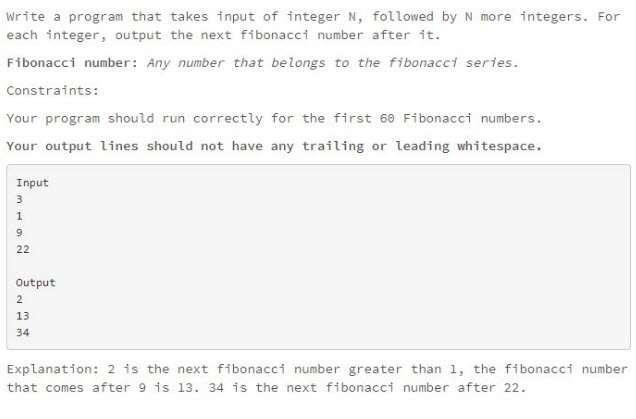# 下一个斐波拉契数（Next Fibonacci Number）

Write a program that takes input of integer N, followed by N more integers.

For each integer, output the next fibonacci number after it.

Fibonacci number: Any number that belongs to the fibonacci series.

Constraints:

Your program should run correctly for the first 69 Fibonacci numbers.

``````Input
3
1
9
22

Output
2
13
34
``````

Explanation: 2 is the next fibonacci number greater than 1, the fibonacci number that comes after 9 is 13. 34 is the next fibonacci number after 22.

## 英文描述## 中文描述

0, 1, 1, 2, 3, 5, 8, 13, 21, 34, 55, 89, 144, 233……（OEIS中的数列A000045）## 测试结果

``````3
1
2019/02/07 20:59:25 DEBUG [com.ossez.codebank.interview.ManNextFibonacciNumber] - 1 Next Fibonacci 
9
2019/02/07 20:59:46 DEBUG [com.ossez.codebank.interview.ManNextFibonacciNumber] - 9 Next Fibonacci 
22
2019/02/07 20:59:49 DEBUG [com.ossez.codebank.interview.ManNextFibonacciNumber] - 22 Next Fibonacci 
``````